nextnano.com  GUI: nextnanomat  Tool: nextnano++  Tool: nextnano³  Tool: nextnano.QCL  Download | Search | Copyright | News | Publications  * password protected nextnano³ software1D SiGe

# nextnano3 - Tutorial

## Simple SiGe structure

Author: Stefan Birner

• Here are the input files:

• `input_file1.in` - simple SiGe structure
• `input_file2.in` - include current
• `input_file3.in` - include quantum models (single-band Schrödinger equation)
• `input_file4.in` - read in raw data and solve 6-band k.p Schrödinger equation

## Now it's time to get started...

(This is a tutorial from 2001. It is more than 17 years old. At that time, nextnano³ was a command line tool. Some parts of the documentation are not really necessary or applicable any more, or are just optional. For backwards compatibility, they are still there but they are shown in light gray color. Some material parameters in the database have changed since then (e.g. band edges). Therefore, one cannot reproduce all figures exactly as they are shown here. Also, the semiconductor structures in this tutorial are a bit artificial. Nevertheless, this tutorial might be helpful to give you a decent introduction into the nextnano³ software.)

1. Step 1: Simple SiGe structure

These input files are needed by the nextnano³ executable:
```- ..\Syntax\database_nn3.in           - ```contains parameters of the materials (don't worry about it now)
``` - ..\Syntax\database_nn3_keywords.val - ```contains the keywords and specifiers for `database_nn3.in`, (no need to worry about it)
```- ..\Syntax\keywords.val              - ```The name of the input file can be written into this file within the keyword``` \$input_filename```.
```                                      - ```Alternatively (recommended): Use nextnanomat or``` -inputfile <filename> ```argument
Apart from this modification` keywords.val ` must not be changed.
`  !------- only to specify input file name ---------!`
```  \$input_filename        optional                   !   input_file1.in        character      optional    !  \$end_input_filename    optional                   !  !------- end only to specify input file name -----!```
`- `In this tutorial, you have four options for the input file:
Start with` input_file1.in `- a simple SiGe structure.
(Later you will use` input_file2.in`,` input_file3.in `and` input_file4.in`.)
2. Open `input_file1.in ` with nextnanomat and read it. There are lots of comments that will explain you what's going on.

If you have any questions regarding the keywords you will find it extremely useful to right-click on your mouse within the nextnanomat text editor. Then a link to the online documentation appears, or have a look at the explanations here.

First we try a simple structure consisting of two regions of SiGe and different alloy concentrations. Since the structure is grown pseudomorphically on Si, the bands are split by strain (different lattice constants, lattice mismatch 4 %).
- No current or quantum states will be calculated.
- It's a 1D simulation.
- Since we only want a classical self-consistent simulation we choose``` flow-scheme = 4```.
This choice means that we are first calculating the built-in potential with the classical nonlinear Poisson equation.
Then the program is calculating a self-consistent solution of the classical Poisson and current equation (if current calculation is specified in the input file - in our case it is not).
3. Output
For the output the destination directories are free to choose, whereas (most of) the file names are fixed and incorporate all information of the file.
- The band structure will be saved into the directory``` band_structure/ ```- The densities will be saved into` densities/`- The strain will be saved into` strain/`
4. Now run the executable by pushing the green button and have a look at the output files with nextnanomat.
Alternatively, you can of course use any other graphics packages as well (e.g. Origin, gnuplot, Matlab, MS Excel, ...).
5. The structure consists of two alloy concentrations of Si1-xGex. The left part has a linear profile (starting with `x=1`, i.e. Ge), the right part is gaussian (with `x=1`, i.e. Ge at the maximum).

```\$material   ...   material-name        = Si(1-x)Ge(x)  ! \$alloy-function   ...   function-name        = linear        !   xalloy-from-to       = 1d0 0d0       ! ```Ge at 0 nm, Si at 500 nm```   vary-from-pos-to-pos = 0d0 500d0     !   ...   function-name        = gaussian-1d   !   gauss-center         = 750d0         !   gauss-width          = 100d0         ! ```sigma```   xalloy-minimum       = 0d0           ! ```Si at minimum```   xalloy-maximum       = 1d0           ! ```Ge at maximum```  ```
6. In the directory``` band_structure/ ```you will find files containing ASCII data (1st column: position in nm, 2nd column: energy in eV).
The meaning of the abbreviations is the following:

` cb1D_Gamma.dat  - `conduction band 1 (Gamma band)
` cb1D_L.dat      - `conduction band 2 (L band)
` cb1D_X.dat      - `conduction band 3 (X band, Here: They are split due to strain) (to be precise: It is Delta for Si and not X.)
` vb1D_hh.dat     - `valence band 1 (heavy hole)
` vb1D_lh.dat     - `valence band 2 (light hole)
` vb1D_so.dat     - `valence band 3 (split-off hole)
` BandEdges1D.dat - `all conduction and valence band edges
` potential.dat   - `electrostatic potential (from Poisson equation)

Both Si and Ge are indirect semiconductors. Bulk Si has its conduction band minimum at the Delta point (500 nm / 1000nm) (we call it X here for simplicity) whereas Ge has its conduction band minimum at the L point (0 nm / 750 nm)).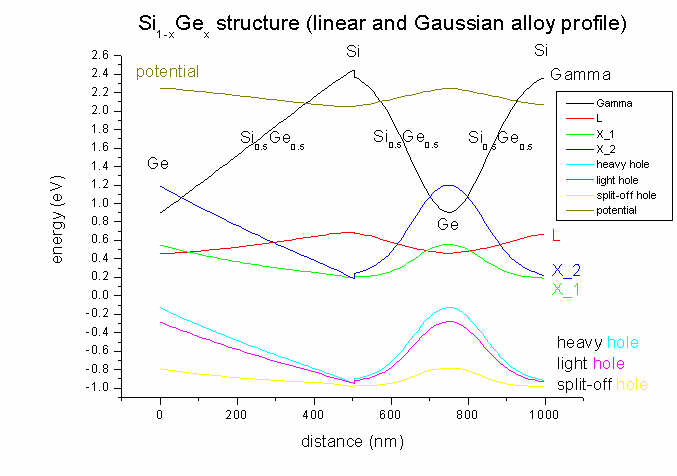7. As the substrate is Si, and the structure is grown pseudomorphically on Si, there will be strain due to the Si-Ge lattice mismatch of 4 % whenever a fraction of Ge is present. In the case of pure Si (at 500 nm) there will be no strain and therefore no splitting of the degenerate X conduction band. In the case of finite strain, the X band degeneracy is lifted, leading to a second X conduction band.
Similarly for the heavy and light hole bands: At 500 nm (Si only, no strain) heavy and light hole are degenerate. This degeneracy is lifted when strain is applied through the incorporation of Ge.
The strain tensor has six dimensionless components (exx, eyy, ezz, exy, exz, eyz) and its values are written to` strain/strain_cr1D.dat`. The ASCII file contains in its columns: position in nm, exx, eyy, ezz, exy, exz, eyz).
In our example file all offdiagonal strain components (exy, exz, eyz) are zero and exx is equal to eyy. The strain has its maximum value when pure Ge is present (0 and 750 nm).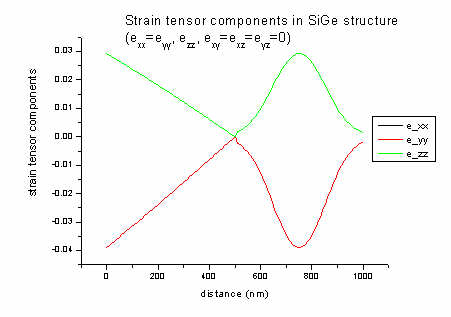In 1D strain can be calculated analytically:
a = lattice constant [nm] (Si: 0.543; Ge: 0.565)
c11, c12 = elastic constants [GPa] (Si: 165.77, 63.93; Ge: 128.53, 48.26)

biaxial strain:                       e|| = exx = eyy = ( asubstrate `-` alayer ) / alayer = ( 0.543 - 0.565 ) / 0.565 = - 0.039    (4 % lattice mismatch)
strain along growth direction: ezz = `-` 2c12 / c11  e|| = `-` 2 * 48/129 * (`-` 0.039) = 0.029

These equations are valid for zinc blende in  growth direction assuming pseudomorphic (=commensurate) epitaxial growth. In this case, shear strain is zero.

8. In the` densities ` directory you will find output for the electron and hole densities of our SiGe structure (and also the space charge):

`density1Del.dat            - `contains electron density
```                             ```(1st column: distance in nm, 2nd column: density in ```1018 cm-3```)
```density1Dhl.dat            - ```contains hole density
`density1DGamma_L_X.dat     - `contains electron density for each band (Gamma, L, X)
`density1Dh_lh_so.dat       - `contains hole density for each band (heavy hole, light hole, split-off hole)
```density1Dpiezo.dat         - ```contains piezoelectric charges
```                             ```(important for III-V semiconductors in  and [N11] growth directions
```                             ==> ```piezoelectric field, see Piezo Tutorial)
`density1Dpyro.dat          - `contains pyroelectric charges
```                             ```(important for pyroelectric materials with wurtzite structure like GaN, AlN and InN
```                             ==> ```spontaneous polarization` ==> `pyroelectric field)
```density1Dspace_charge.dat  - ```contains the space charge density (here: no donors and acceptors present)
```                             = -``` electron density + hole density + ionized donors `-` ionized acceptors
`interface_densitites1D.txt - `contains information about the piezo and pyroelectric charge densities at
```                             ```interfaces (position in nm of interface and 2D interfacial number density in units of``` [1012 cm-2]```.

The electron/hole density output will be given in units of` [````1018 cm-3````]`. These units are the same for 1D, 2D and 3D simulations.

Note: The pictures shown below were created with a previous version of nextnano³. Meanwhile the parameters in the database have changed (as well as some bugs were fixed). So the pictures do not necessarily have to coincide with the results obtained from the current version of nextnano³. Especially in this example, the density depends very critically on tiny changes in the database parameters.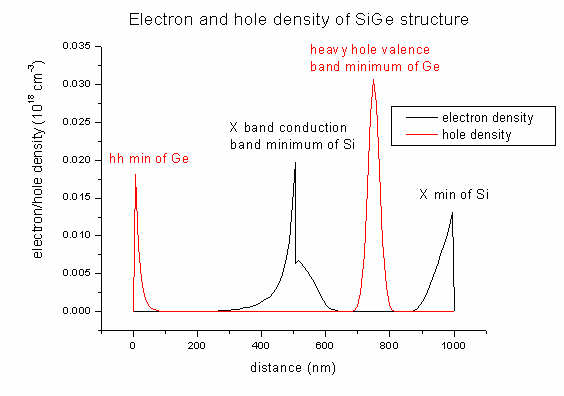The space charge in our case looks like this (sum of hole minus electron density).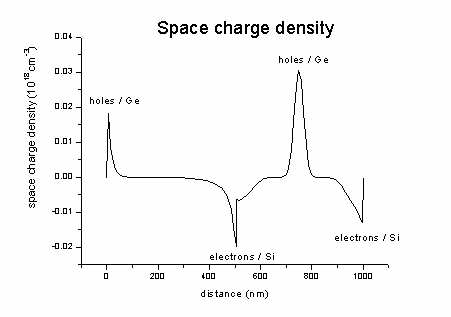9. Step 2: Include current
Now let's move on to` input_file2.in`.
10. Now we try to put some dopants into our structure and apply some voltage to see if there is a current.
For dopants we need:
- doping functions
- impurity parameters
For the current we need:
- contacts (Poisson boundary conditions)
- current regions and clusters
- current models
For the contacts we use two additional regions of material Metal, located at the edges of the simulation region.
First run without applied voltage and after that try with different applied voltages but not larger than 0.1 V. (For larger voltages you should solve the equations step wise` ==> ` voltage sweep.)
In order to see the effects of doping, we take simpler alloy functions, namely constant alloys, i.e. our structure consists of Si only.
11. Doping
First you have to specify a doping profile. This can be done by the keyword``` \$doping-function```.``` ```The doping profile is independent of the regions specified before. The function is applied to the region given by the specifier` only-region`.

The first profile is a constant doping with concentration``` 1d1 * 1018/cm3 (1d1 = 1*101 = 10)```.

The second profile is a well with double Gaussian walls. The walls are centered at parameter `center` and the slope of the walls is given by` width`.

The doping concentration is at the position specified by the specifier``` position ```(optional for constant doping profile).
The function is normalized such that the result is doping concentration at position` position`.

The impurity number specifies the kind of impurities used in the profile. Consider the degeneracy factor of the impurity levels (2 for donors and 4 for acceptors). For details please confer``` \$impurity-parameters```.

We take a Si structure with constant n-type doping between 100 nm and 200 nm (1*1019 cm-3) and a p-type doping well (with Gaussian walls centered at 600 and 800 nm (1*1018 cm-3) ).
12. Current
In order to apply any voltage to the device you have to define contacts. This is done by the Poisson boundary conditions. There are mainly two different kinds:
- `Schottky` (implies a Schottky barrier, can be used to simulate surface states)
- `ohmic` (no barrier)

These Poisson clusters are assigned to the region-clusters that should serve as the contacts. (Here they consist of the material Metal but the material name can be arbitrary as the contacts are not included in the Poisson,Schrödinger or current equation.).

The voltage difference should not be too large because of convergence issues. Larger voltage should be calculated stepwise using voltage-sweep or adjusting them manually by reading in the previous potential and Fermi levels.

To calculate the current flow due to the applied voltage in the Poisson boundary conditions, one has to specify certain current regions and clusters analogous to the material and quantum clusters. On each cluster one can apply a certain current model (so far only simple drift-diffusion but there is more to come).

It is possible to specify different regions for different current models but since so far only one model is implemented, you can skip the current region keyword. By not specifying any region, the whole simulation area is region number 1. Then a current cluster containing this region 1 has to be specified.
Mobility parameters for all materials contained in the current cluster have to be present either in the database or in the input file. For now, also for the metal contacts, mobility parameters have to exist although they are not used in the calculation.
13. Output
- The band structure will be saved into the directory``` band_structure/ ```- The densities will be saved into``` densities/ ```- The strain will be saved into``` strain/ ```- The current will be saved into``` current/ ```Note: Please add the specifier``` IV-curve-out = yes ```to the keyword``` \$output-current-data ```in order to print out current data (I-V characteristics). (The file``` current.dat ```will only contain zeros.)
```  \$output-current-data    ...    IV-curve-out = yes```
14. Now run the executable and have a look at the output files.
15. Current is set to` -`0.1 V.
` applied-voltage  =  -0.1d0  ! [V]`
16. The band structure (conduction and valence bands) and the potential looks like this: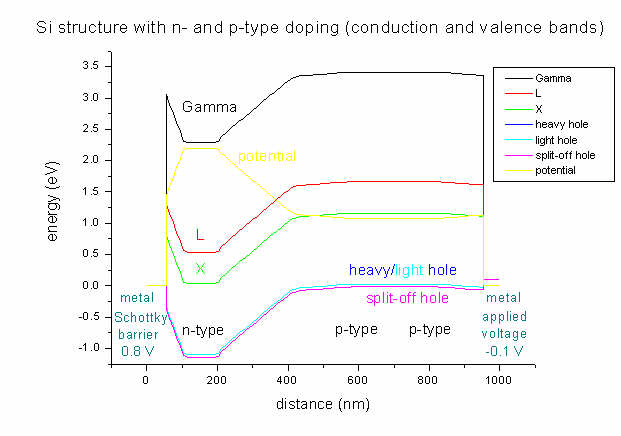As boundary conditions, on the left side a Schottky barrier of 0.8 V is assumed whereas on the right side a voltage of `-`0.1 V is applied. As can be seen, the lowest conduction band in Si is the X band. Strain is zero in this case (Si pseudomorph on Si), thus heavy and light hole bands are degenerate and there is no X band splitting (compare` input_file1.in`). It can clearly be seen that the area with heavy n-type doping (between 100 and 200 nm) "pulls down" the conduction band. The electrostatic potential (yellow line) has its highest value in the n-type region and its lowest in the p-type region (between 600 and 800 nm).
17. For comparison only, here's the band structure for the same doping profile but with Schottky barrier = 0 V and applied voltage = 0 V.18. Now let's go back to our ``` input_file2.in```.
Here we plot the electron and hole densities (and also the space charge density; units 1018 cm-3):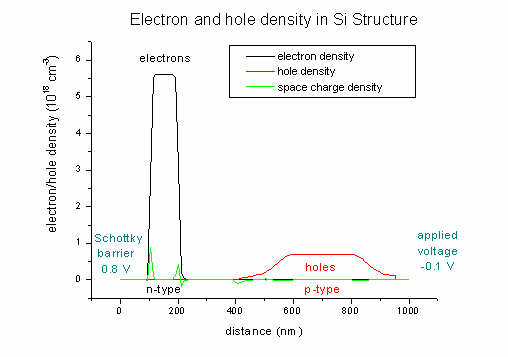19. The n and p mobilities:
The mobility output` mobility1D.dat `is on a different grid (as well as the drift velocities and the current)` -> `see material grid vs. physical grid for details).

Electron (black line) and hole mobilities (red line) in Si structure (applied voltage: `-`0.1 V)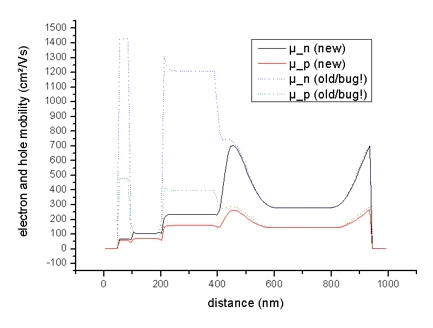(The dotted lines should be ignored!)

20. The diagrams of the drift velocity vd,n (electrons) and vd,p (holes):
`drift_velocity1D.dat - `units: cm/s

Drift velocities of electrons (black line) and holes (red line) in Si structure  (applied voltage: `-`0.1 V)21. The quasi-Fermi levels for n (electrons) and p (holes):
`FermiLevel1D.dat - `quasi-Fermi level for electrons and for holes
The difference between left and right boundaries is the applied voltage of `-`0.1 V.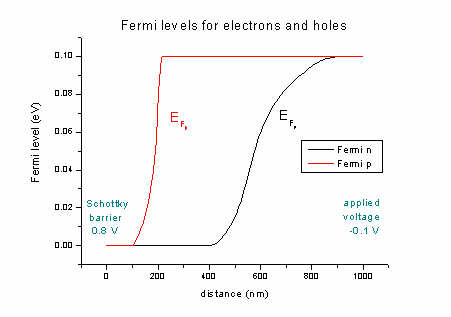If no voltage is applied the quasi-Fermi levels are constant (no current flow). Our ansatz for the current: It is proportional to the mobility and to the gradient of the quasi-Fermi level (drift-diffusion model).
More details for the current output can be found here.

`currentD.dat`
The total current that is flowing is jtot = jel + jhl = 2.45 * 10-8 A/m2 + 0.24 * 10-8 A/m2 = 2.70 * 10-8 A/m2.
22. Now, let's switch current off.
``` applied-voltage = 0.0d0 ! [V] ```The quasi-Fermi levels are constant (zero) (`FermiLevel1D.dat`).
The drift velocities are zero (`drift_velocity1D.dat`).
Obviously, the current flow is zero (`currentD.dat`).
23. If we had no doping, the effect of an applied voltage of `-`0.1 V for the quasi-Fermi level EF,n of the electrons would be (same for EF,p):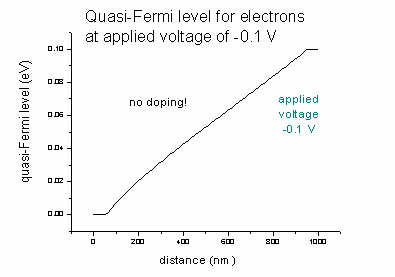24. Step 3: Include quantum models (1-band Schrödinger equation)
Now let's include quantum models. Have a look at``` input_file3.in```. So far we only used classical electrostatics which is fast but not very interesting. If you have small structures where quantization effects are no longer negligible then you might include either 1-band Schrödinger equations, or multi-band k.p into the simulation.
1-band:  Each band (Gamma, L, X, heavy, light, split-off hole) is treated independently.
6-band k.p: The three valence bands (heavy, light, split-off hole) are coupled but electron bands are treated independently of them.
8-band k.p: The three valence bands and the Gamma band are coupled.

For a quick check if quantum mechanics does change anything, 1-band Schrödinger is certainly enough. But if you need a detailed description of the valence band, k.p is necessary.

Since k.p takes some time, we try to get k.p results for a nonequilibrium case in a two step approach here:
First (`input_file3.in`), we calculate potentials and quasi-Fermi levels with 1-band Schrödinger. We put out this data into the directory``` raw_data/```.
Secondly (`input_file4.in`), we read in Fermi levels and potentials from` raw_data/ `and calculate k.p eigenstates.

We change the structure to a SiGe quantum well with 20 nm width and skip all doping in order to keep things simple.
Si / Si0.7Ge0.3 / Si
``` \$regions```
...
``` region-number = 3   base-geometry = line   region-priority = 2 ```
Here we specified region number 3 with a higher priority than region 2 which means that it is on top of the lower priority. Region 3 is going to be our well.

`\$grid-specification`
We take a higher density of nodes in the well region, because this will determine the quality of our wave functions. There are also two extra grid lines at` 480d0 ` and``` 540d0 ``` which are the boundaries of the quantum region. The quantum region should be larger than the well and should have dense gridlines.
25. Since we want a quantum solution with current, we take ``` flow-scheme = 2```.
This means that we are first calculating the potential with the classical nonlinear Poisson equation. With this starting value, the program is calculating the built-in potential with the nonlinear Poisson equation (and specified densities). After that, the program is determining the self-consistent solution of the Schrödinger, Poisson and current equations.
26. For quantum solutions you have to define quantum regions and clusters on which certain quantum models are applied. The syntax of the quantum regions and clusters is the same as for current and material regions. The model specifies the kind of equation that has to be solved in the certain cluster.
For the first step we want to solve the 1-band Schrödinger equation for conduction band 2 (L band) and valence bands 1 2 3 (heavy hole, light hole and split-off hole).
For each band we want 10 eigenvalues which is specified in '```number-of-eigenvalues-per-band = 10 10 10```'

As boundary conditions for the holes we take` ``Dirichlet`. Dirichlet boundary conditions mean that the wave function is zero at the boundaries.
As boundary conditions for the electrons we take` Neumann`. Neumann boundary conditions mean that the derivative of the wave function with respect ot position is constant at the boundaries (dpsi/dz = 0).
27. Output
- The band structure will be saved into the directory``` band_structure/ ```- The densities will be saved into``` densities/ ```- The strain will be saved into``` strain/ ```- The current will be saved into``` current/ ```- Raw data will be saved into` raw_data/` (file names : '`fermi_store1D.raw`', `'potentials_store1D.raw`').``` ```This output is unformated data which can be read in again by e.g. ``` flow-scheme = 3```.

For the 1-band Schrödinger solutions a file name looks like: '`cb002_qc001_sg001_deg001_dir.dat`'
In this file the eigenvalues and eigenfunctions are stored for the parameters:
`cb002`:   conduction band number 2 (1 = Gamma, 2 = L, 3 = X)
`qc001`:   quantum region cluster 1
`sg001`:   Schrödinger equation number 1
`deg001`: number of subsolution (degeneracy)
`dir`:       boundary condition (`dir` = Dirichlet, `neu` = Neumann)
The file '`sg-definition1D.txt`' provides some information about the structure of the 1-band solutions.
The meaning of
`sg:`
For different band energies, different Schrödinger equations have to be solved. These are numbered by `sg (sg001, sg002, ...)`.``` deg:```
For equal energies but different masses, again, different equations have to be solved, which are then numbered by `deg (deg001, deg002, ...)`.
These files will be stored into directory` Schroedinger_1band/`.``` ```Now run the executable and have a look at the output files.
28. Here we plot the band profile and potential of our Si / SiGe / Si structure. The 20 nm Si0.7Ge0.3 quantum well is between 500 and 520 nm. The applied voltage is 0.1 V. Due to strain in the quantum well area the X band is split (500-520 nm).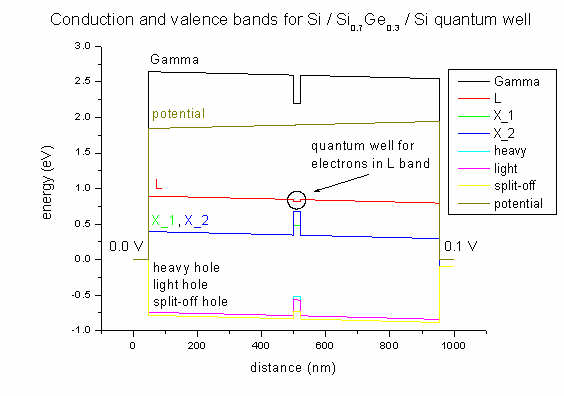Strain is zero except for the exx, eyy and ezz strain tensor components inside the Si0.7Ge0.3 quantum well region. This leads to a splitting of the X conduction band. This structure was grown pseudomorphically on Si. So the SiGe alloy is strained pseudomorphically with respect to this Si substrate.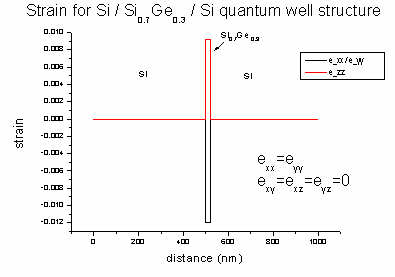29. We are interested in the eigenvalues (energies) and eigenfunctions (Psi2) of the electrons in the L band inside the quantum well. We can see that there are 5 bound states inside the quantum well. (They are the same for both Neumann and Dirichlet boundary conditions. However, delocalized states (here: (6)) differ for Neumann and Dirichlet). The eigenenergies are given in eV and the electron wave functions (squared) are plotted.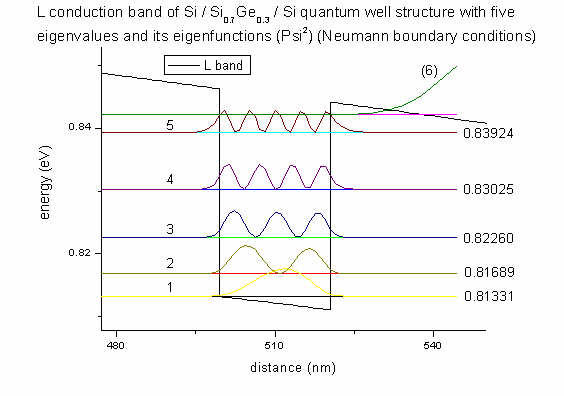All this information is given in the file
`  Schroedinger_1band/cb002_qc001_sg001_deg001_neu_shift.dat ` (L band, Neumann)
which contains the eigenvalues and Psi2. The suffix``` shift ```indicates that the square of the wave functions is shifted by its eigenvalue. Then the wave functions can be plotted much nicer into the band edge plot.
The columns are ordered as follows:
position (nm)      eigenvalue 1 (eV)      ...      eigenvalue 10      (eigenfunction 1)2      ...    (eigenfunction 10)2
More information can be found in the file``` Schroedinger_1band/sg_info.txt ```and in``` \$output-1-band-schroedinger```
The band edge profile of the L conduction band profile was taken from the file``` cb1D_L.dat```.
30. The Fermi levels for electrons (n) and holes (p) of the Si / SiGe / Si quantum well structure (applied voltage 0.1 V):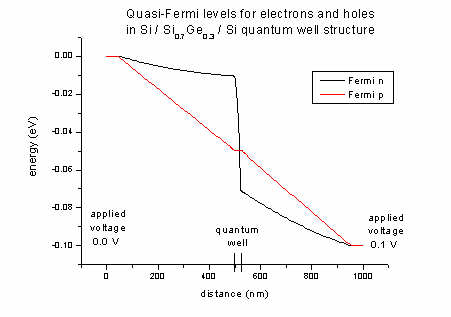31. The mobility of electrons and holes of the Si / SiGe / Si quantum well structure (applied voltage 0.1 V):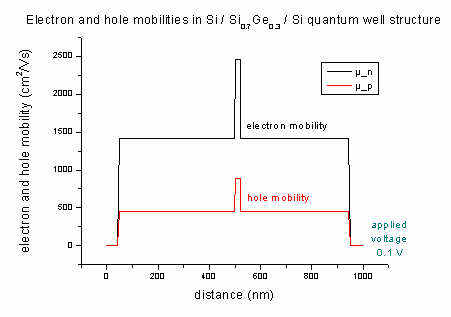32. The density of electrons and holes of the Si / SiGe / Si quantum well (applied voltage 0.1 V):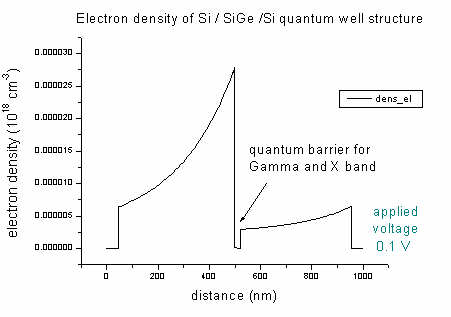The Si0.7Ge0.3 alloy acts as a quantum well for heavy, light and split-off holes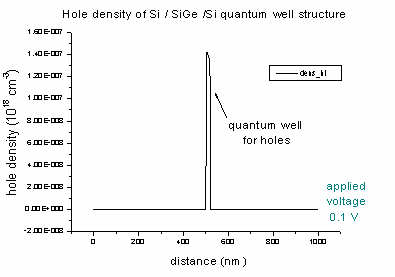33. Step 4: Read in data and solve k.p
Now we move on to``` input_file4.in```.
In the third file, we solved the current problem with 1-band Schrödinger and saved the data (electrostatic potential and Fermi levels). Now we read in the electrostatic potential and the Fermi levels again in order to get the k.p wave functions. The input file has to remain almost the same, just the quantum model is changed from` effective-mass ` to` 6x6kp`.
Also the `flow-scheme` is now number` 3 `(read in raw data and calculate eigenstates).
So let's see if it works...
34. Since we want to read in raw data and solve for eigenfunctions, we take``` flow-scheme = 3 ```which means that the built-in potential, the potential and the quasi Fermi levels, which completely determine the system, will be read in. For this potential, the specified eigenstates will then be calculated.
We have to specify from which directory the raw data has to be read in:
```  raw-directory-in   = raw_data/ ```We also need to set the flags to` yes ` to read in raw data:
```  raw-potential-in    = yes   raw-fermi-levels-in = yes```
35. Output k.p data
Possible outputs for k.p

Bulk k.p dispersion
- Plots dispersion of bulk k.p Hamiltonian including strain effects.
- Filename: '`bulk_6x6kp_dispersion_000_kxkykz.dat`'
See tutorial: k.p dispersion in bulk GaAs (strained / unstrained)

k|| dispersion
- Plots dispersion perpendicular to quantization axes for eigenvalues from min to max.
- Result: 2D plot in AVS/Express format.
- Data files for 1st subband: '```kpar1D_disp_00_10_hl_6x6kp_ev_001_2Dplot.dat / .coord / .fld```'

Eigenvalues, eigenfunctions
- Filename: `kp_6x6eigenvalues_qc001_kpar0001_Kz001_dir.dat`
- Specifier:
 `kp_8x8, kp_6x6` kind of k.p solved `_el, _hl` electrons/holes `eigenvalues, psi_squared` eigenvalues, eigenfunctions squared `_qc001` quantum cluster 1 `_ev001` eigenvalue 1 `_kpar001` number of k|| = 1 `_Kz001` number of superlattice vector = 1 `_dir, _neu` boundary conditions Dirichlet or Neumann

More information: `\$output-kp-data`

36. Output
- The band structure will be saved into the directory``` band_structure/ ```- The densities will be saved into``` densities/ ```- The strain will be saved into``` strain/ ```- The current will be saved into``` current/ ```- Raw data will be saved into``` raw_data/ ```- k.p data will be saved into` kp_data/`
37. Now run the executable and have a look at the output files.
This time the calculation takes more time (maybe even some minutes).
38. In the k.p directory` kp_data/ `you will find for each eigenvalue 3 files:
- eigenvalue
position in space [nm], eigenvalue
- eigenfunction squared
position in space [nm], Psi2 (sum of components), squares of components of k.p vector (8 in case of 8-band k.p / 6 for 6-band k.p)

In our case for 6-band k.p each file contains information about the holes ordered in the basis
` | x`+` >, | y`+` >, | z`+``` >, | x- >, | y- >, | z- >```

`| x >, | y >, | z > `correspond to x, y, z of the calculation system.
`+ `(spin up)` `and` - `(spin down)` `correspond to the spin projection along the z axis of the crystal system.
Spin up and spin down eigenvalues are degenerate in this example.

Here we plot the energies and hole wave functions (squared) of our 6-band k.p calculation. We have twofold spin degeneracy, thus we only plot spin up values. The 9th eigenvalue (and of course also the 10th) has light hole character whereas the first eight eigenvalues have heavy hole character.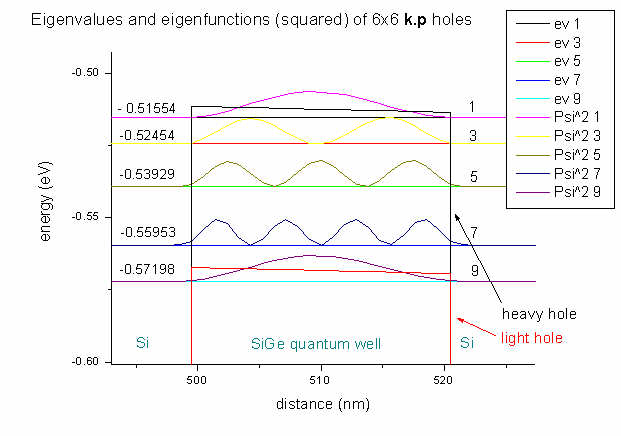The arrows point to the heavy hole and light hole band edges.

• Please help us to improve our tutorial! Send comments to``` support [at] nextnano.com```.